# Using the PMT, PPMT, IPMT and ABS functions to create a Mortgage Calculator in Excel 2007 and Excel 2010

## Introduction

Welcome to my latest two part hub on Excel 2007 and Excel 2010. In Part One today, I will discuss how to create a simple mortgage calculator using Excel. To achieve this, I will use three Excel functions

1. PMT which will be used to calculate the total repayment amount
2. PPMT will be used to calculate the amount of principal repaid
3. IPMT to calculate the interest paid

My mortgage calculator will be able to calculate the repayments, interest and principal paid for either a repayment or an interest only mortgage paid either quarterly or monthly.

• In the background, I will also be using the ABS function (this function returns the absolute value of a number) which I use to remove the – sign from a number.
• To allow me to control input from the user of the calculator, I am also using Combo boxes (the reasons behind this choice will become evident later on in this hub).
• To convert the results of the Combo boxes into numbers the PMT, PPMT and IPMT functions can use, I will be using IF statements

Once the calculator is completed, the end result will be similar to mine below.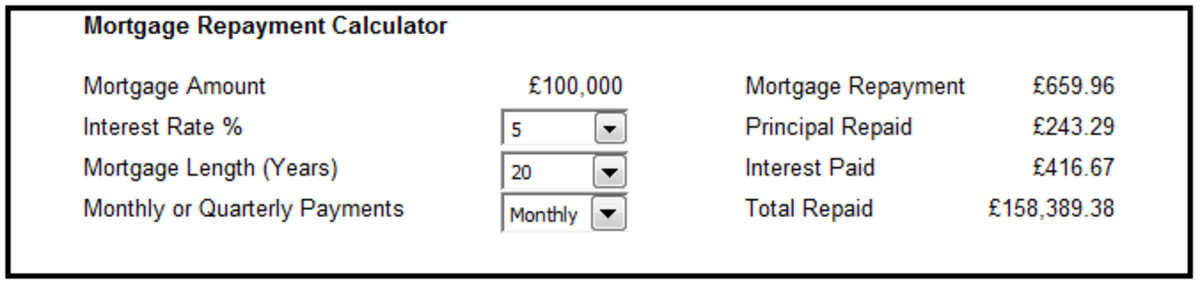Example of a completed Mortgage Calculator, created using Excel 2007 and Excel 2010.http://robbiecwilson.hubpages.com/

In Part Two, I will create an Amortization schedule which allows you to determine how much interest you pay each time you make a payment as well as calculating the effect of an overpayment. That can be found here:

http://robbiecwilson.hubpages.com/hub/Creating-an-Amortisation-loan-or-mortgage-schedule-using-Excel-2007-and-Excel-2010

## Using PMT to calculate the repayment amount in Excel 2007 and Excel 2010

To begin with, we need to calculate the total repayment per month (or quarter). To do this, we us the PMT function.

The PMT functions syntax is as follows

Note: I have each variable on a separate line only for readability.

=PMT(Interest Rate / The number of repayments per year,

The number of repayments per year multiplied by the number of years,

The total amount of the mortgage,

Final value of the mortgage (if this is omitted then it is assumed to be 0),

Type which indicates when payments are made (0 or omitted assumes that interest is paid at the end of the period, 1 indicates it is paid at the beginning)

The figure below shows an example of the PMT function with the majority of the syntax replaced with the numbers used for simplicity.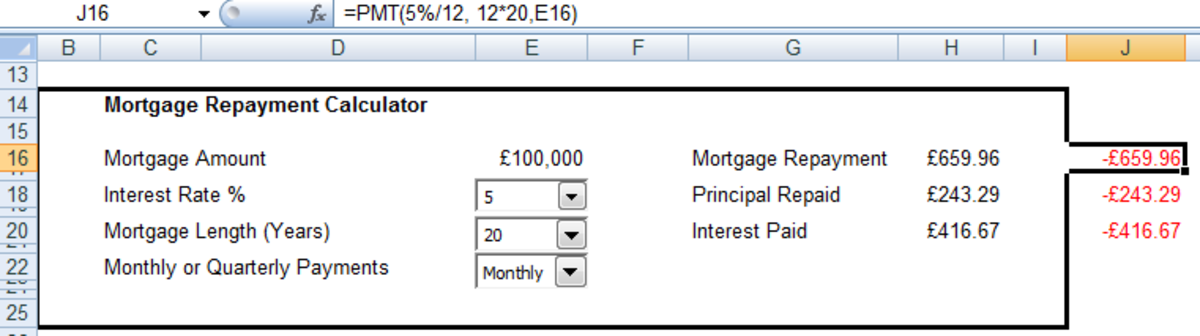Example of the syntax of the PMT function used to calculate the overall mortgage repayment in Excel 2007 and Excel 2010. http://robbiecwilson.hubpages.com/
Scroll to Continue

NOTE: The cell containing the Interest Rate must have a % sign in the cell. If you do not include one, you will get an answer but it will be nonsensical. This is the reason that a combo box is used for the Interest Rate, to ensure that a % sign is used. Otherwise I run the risk of a % sign not being used and the calculations being inaccurate.

An example of a completed formula using the PMT function:

=PMT(Q2/12, L2*20,E16,0,0)

Q2 = 5.00% < Interest Rate >

L2 = 12 < Payment periods per year >

E16 = £100,000 < Mortgage Value >

0 = < Final value of Mortgage = 0 >

0 = < Interest is paid at the end of the month >

NOTE: The result Excel gives you is a negative number. To turn this into a number we can use, we need to use the ABS function. To use the ABS function, simply enter the following into the cell you are looking to convert from a negative

=ABS(J16)

The figure below shows the result of the PMT function in red and the value after ABS has been used in black.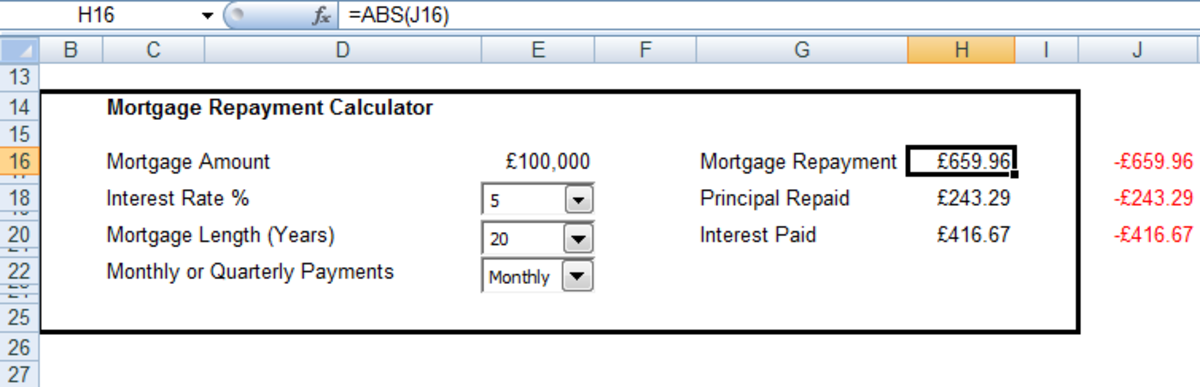Using the ABS function to make a negative number positive in Excel 2007 and Excel 2010.http://robbiecwilson.hubpages.com/

## Using PPMT to calculate the amount of Principal paid using Excel 2007 and Excel 2010

The PPMT function is very similar to the PMT function in its syntax. The key difference is the addition of the period in the mortgage we are examining (in this case the first month).

Note: I have each variable on a separate line again only for readability.

=PPMT(Interest Rate / The number of repayments per year,

The period we are looking at (in this case the first month),

The number of repayments per year multiplied by the number of years,

The total amount of the mortgage,

Final value of the mortgage (if this is omitted then it is assumed to be 0),

Type which indicates when payments are made (0 or omitted assumes that interest is paid at the end of the period, 1 indicates it is paid at the beginning)

An example of a completed formula using the PPMT function:

=PPMT(Q2/L2,1,L2*20,E16)

Q2 = 5.00% < Interest Rate >

L2 = 12 < Payment periods per year >

1 = < the first month of the mortgage >

E16 = £100,000 < Mortgage Value >

0 = < Final value of Mortgage = 0 >

0 = < Interest is paid at the end of the month >

The figure below shows an example of the PPMT function with the majority of the syntax replaced with the numbers used for simplicity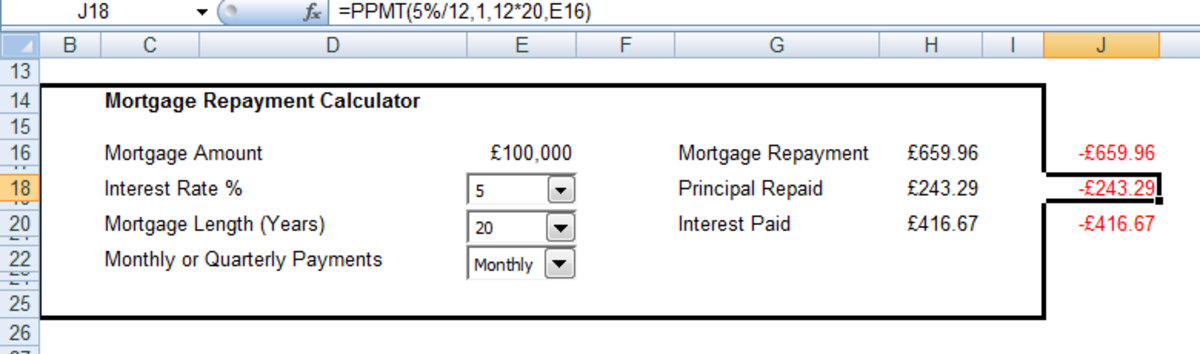Syntax of the PPMT function used to calculate the Principal paid on a mortgage in Excel 2007 and Excel 2010.http://robbiecwilson.hubpages.com/

## Using IPMT to calculate the amount of Interest paid in Excel 2007 and Excel 2010

The IPMT function is identical to PPMT in its format. The difference being that it calculates interest paid rather than principal paid.

## Using Combo Boxes in the Mortgage calculator in Excel 2007 and Excel 2010

To ensure that the input from the user of my calculator is exactly what I want, I decided to use Combo Boxes with drop downs, rather than allowing free typing for the Interest Rate, the Mortgage Length and the whether the Payments were monthly or quarterly.

Combo boxes return a value based on which option was selected, so for Payments:

Monthly = 1

Quarterly = 2

We need to convert this to a number we can use in our PMT, PPMT and IPMT formulas.

To do this, I will use IF statements. In this instance our IF statement is quite straightforward.

=IF(K2=1,"12",IF(K2=2,"4"))

This converts the number returned by the Combo box into the numbers our functions need.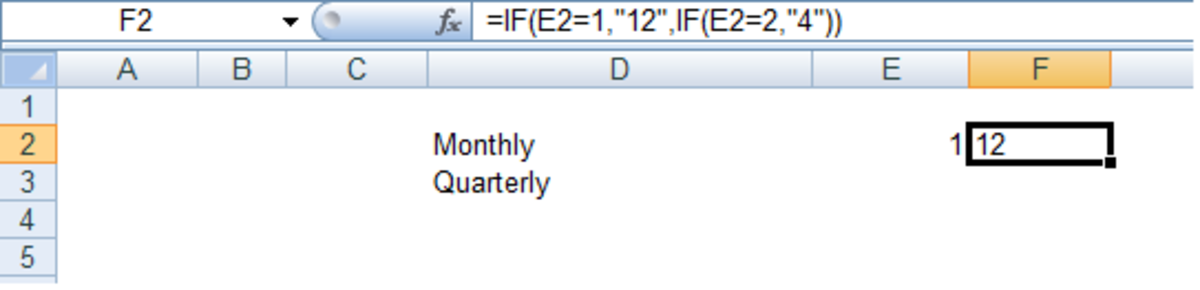Using IF statements to convert the contents of a cell in Excel 2007 and Excel 2010.http://robbiecwilson.hubpages.com/

Note: The number in K2 is the number returned by the Combo box. The contents of D2 and D3 are used by Excel 2007 or Excel 2010 to create the options for the Combo box for Monthly or Quarterly Payments.

I have a hub that covers creating and using Combo boxes in far more detail that can also be found here:

http://robbiecwilson.hubpages.com/hub/Configuring-and-Using-Combo-boxes-in-Excel-2007

I also have a hub that goes into greater depth on the IF statement that can be found here:

http://robbiecwilson.hubpages.com/hub/Using-the-IF-and-IFERROR-functions-as-well-as-the-logical-functions-AND-OR-and-NOT-in-Excel-2007-and-Excel-2010

In today’s hub, we have created a mortgage calculator using the:

• PMT function to calculate the overall repayments as well as
• PPMT to calculate the principal paid and
• IPMT to calculate the interest portion
• ABS to convert a negative number to a positive one and finally
• We used Combo boxes and IF statements to control user input and to convert the results from the Combo boxes into the numbers used by the formulas we created.

In Part Two of my mortgage calculator hub, I will be looking at creating an amortization schedule for a mortgage. I will be investigating two methods,

• The first is to create on using the formulas that we have used in part one and
• The second way is to use a built in template that Excel 2007 provides.

That hub can be found here:

http://robbiecwilson.hubpages.com/hub/Creating-an-Amortisation-loan-or-mortgage-schedule-using-Excel-2007-and-Excel-2010

Both Excel 2007 and Excel 2010 are very powerful pieces of software and have the ability to take already potent functions and combine them with a number of others to create something very useful which makes Excel continually fascinating to use. I do hope that you have enjoyed reading this hub and that you have found something useful for you in amongst the functions I have used today to create my mortgage calculator. Many thanks for reading, please feel free to leave any comments you may have below.